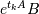# scipy.sparse.linalg.expm_multiply¶

scipy.sparse.linalg.expm_multiply(A, B, start=None, stop=None, num=None, endpoint=None)[source]

Compute the action of the matrix exponential of A on B.

Parameters: A : transposable linear operator The operator whose exponential is of interest. B : ndarray The matrix or vector to be multiplied by the matrix exponential of A. start : scalar, optional The starting time point of the sequence. stop : scalar, optional The end time point of the sequence, unless endpoint is set to False. In that case, the sequence consists of all but the last of num + 1 evenly spaced time points, so that stop is excluded. Note that the step size changes when endpoint is False. num : int, optional Number of time points to use. endpoint : bool, optional If True, stop is the last time point. Otherwise, it is not included. expm_A_B : ndarray The result of the action.

Notes

The optional arguments defining the sequence of evenly spaced time points are compatible with the arguments of numpy.linspace.

The output ndarray shape is somewhat complicated so I explain it here. The ndim of the output could be either 1, 2, or 3. It would be 1 if you are computing the expm action on a single vector at a single time point. It would be 2 if you are computing the expm action on a vector at multiple time points, or if you are computing the expm action on a matrix at a single time point. It would be 3 if you want the action on a matrix with multiple columns at multiple time points. If multiple time points are requested, expm_A_B will always be the action of the expm at the first time point, regardless of whether the action is on a vector or a matrix.

References

 [R185] Awad H. Al-Mohy and Nicholas J. Higham (2011) “Computing the Action of the Matrix Exponential, with an Application to Exponential Integrators.” SIAM Journal on Scientific Computing, 33 (2). pp. 488-511. ISSN 1064-8275 http://eprints.ma.man.ac.uk/1591/
 [R186] Nicholas J. Higham and Awad H. Al-Mohy (2010) “Computing Matrix Functions.” Acta Numerica, 19. 159-208. ISSN 0962-4929 http://eprints.ma.man.ac.uk/1451/

#### Previous topic

scipy.sparse.linalg.expm

#### Next topic

scipy.sparse.linalg.onenormest